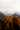# What is a Data Structure?

#### Data structures are collections of values, the relationships among them, and the functions or operations that can be applied to the data.

Most of the data structures do not come 'for free' in JavaScript, we will need to implement our own with ES2015 Class syntax.

# Simple Class Example

``````// upon instantiating a new Student object, firstName and
// lastName must be passed in, else they will be undefined.
class Student {
constructor (firstName, lastName, year) {
this.firstName = firstName;
this.lastName = lastName;
// shows that the argument names don't need to match
// the class variable names.  The student object will
// have a student.grade value, NOT a student.year.
this.tardies = 0;
this.scores = [];
}
// instance method:
fullName(){
return `My full name is \${this.firstName} \${this.lastName}`
}
markLate() {
this.tardies += 1;
return `\${this.firstName} \${this.lastName} has been late \${this.tardies} times `
}
// while you could access the scores array on a Student instance directly,
// we assume that a good developer will interact with this provided interface.
this.scores.push(score);
return this.scores;
}
calculateAverage() {
let sum = this.scores.reduce((a, b) => {
return a + b
});
return sum / this.scores.length;
}
// Class Method (note the capital first letter)
static EnrollStudents (...students) {
// send emails to all students in array of student
// instances passed into function.
return 'enrolling students'
}

}``````

### To create an instance of Student:

``````let firstStudent = new Student("David", "Attenborough", 0)
let secondStudent = new Student("Axl", "Rose", 3)``````

#### Calling an instance method

``````firstStudent.fullName()
// => My full name is David Attenborough

``Student.EnrollStudents([firstStudent, secondStudent])``
``Point.distance(pointA, pointB)``